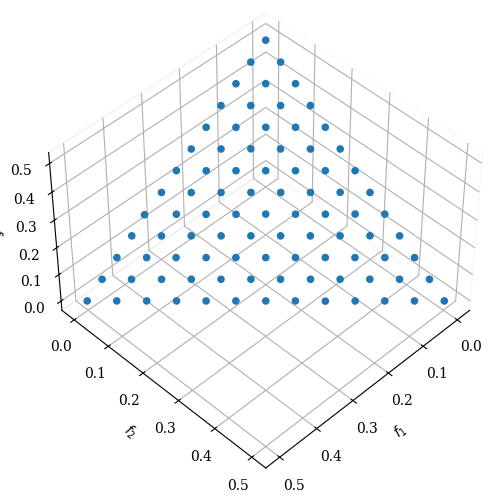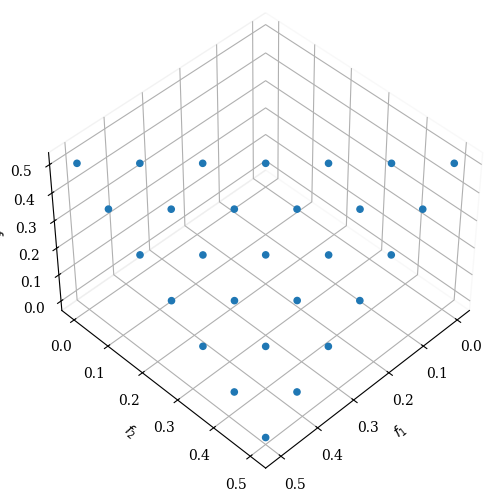Version: 0.5.0

# NSGA-III¶

The algorithm is implemented in base of  . Implementation details of this algorithm can be found in . NSGA-III is based on Reference Directions which need to be provided when the algorithm is initialized.

The survival, first, the non-dominated sorting is done as in NSGA-II.Second, from the splitting front, some solutions need to be selected. NSGA-III fills up the underrepresented reference direction first. If the reference direction does not have any solution assigned, then the solution with the smallest perpendicular distance in the normalized objective space is surviving. In case a second solution for this reference line is added, it is assigned randomly.Thus, when this algorithm converges, each reference line seeks to find a good representative non-dominated solution.

## Example¶

:

from pymoo.algorithms.moo.nsga3 import NSGA3
from pymoo.factory import get_problem, get_reference_directions
from pymoo.optimize import minimize
from pymoo.visualization.scatter import Scatter

# create the reference directions to be used for the optimization
ref_dirs = get_reference_directions("das-dennis", 3, n_partitions=12)

# create the algorithm object
algorithm = NSGA3(pop_size=92,
ref_dirs=ref_dirs)

# execute the optimization
res = minimize(get_problem("dtlz1"),
algorithm,
seed=1,
termination=('n_gen', 600))


:

<pymoo.visualization.scatter.Scatter at 0x7fd6777dd0a0>:

res = minimize(get_problem("dtlz1^-1"),
algorithm,
seed=1,
termination=('n_gen', 600))


:

<pymoo.visualization.scatter.Scatter at 0x7fd675654580>## API¶

class pymoo.algorithms.moo.nsga3.NSGA3(self, ref_dirs, pop_size=None, sampling=FloatRandomSampling(), selection=TournamentSelection(func_comp=comp_by_cv_then_random), crossover=SimulatedBinaryCrossover(eta=30, prob=1.0), mutation=PolynomialMutation(eta=20, prob=None), eliminate_duplicates=True, n_offsprings=None, display=MultiObjectiveDisplay(), **kwargs)
Parameters
ref_dirsnumpy.array

The reference direction that should be used during the optimization. Each row represents a reference line and each column a variable.

pop_sizeint (default = None)

By default the population size is set to None which means that it will be equal to the number of reference line. However, if desired this can be overwritten by providing a positive number.

sampling

The sampling process defines the initial set of solutions which are the starting point of the optimization algorithm. Here, you have three different options by passing

(i) A Sampling implementation which is an implementation of a random sampling method.

(ii) A Population object containing the variables to be evaluated initially OR already evaluated solutions (F needs to be set in this case).

(iii) Pass a two dimensional numpy.array with (n_individuals, n_var) which contains the variable space values for each individual.

selectionSelection

This object defines the mating selection to be used. In an evolutionary algorithm each generation parents need to be selected to produce new offsprings using different recombination and mutation operators. Different strategies for selecting parents are possible e.g. selecting them just randomly, only in the neighborhood, using a tournament selection to introduce some selection pressure, …

crossoverCrossover

The crossover has the purpose of create offsprings during the evolution. After the mating selection the parents are passed to the crossover operator which will dependent on the implementation create a different number of offsprings.

mutationMutation

Some genetic algorithms rely only on the mutation operation. However, it has shown that increases the performance to perform a mutation after creating the offsprings through crossover as well. Usually the mutation operator needs to be initialized with a probability to be executed. Having a high probability of mutation will most of the time increase the diversity in the population.

eliminate_duplicatesbool

The genetic algorithm implementation has a built in feature that eliminates duplicates after merging the parent and the offspring population. If there are duplicates with respect to the current population or in the offsprings itself they are removed and the mating process is repeated to fill up the offsprings until the desired number of unique offsprings is met.

n_offspringsint (default: None)

Number of offspring that are created through mating. By default n_offsprings=None which sets the number of offsprings equal to the population size. By setting n_offsprings=1 a, so called, steady-state version of an algorithm can be achieved.